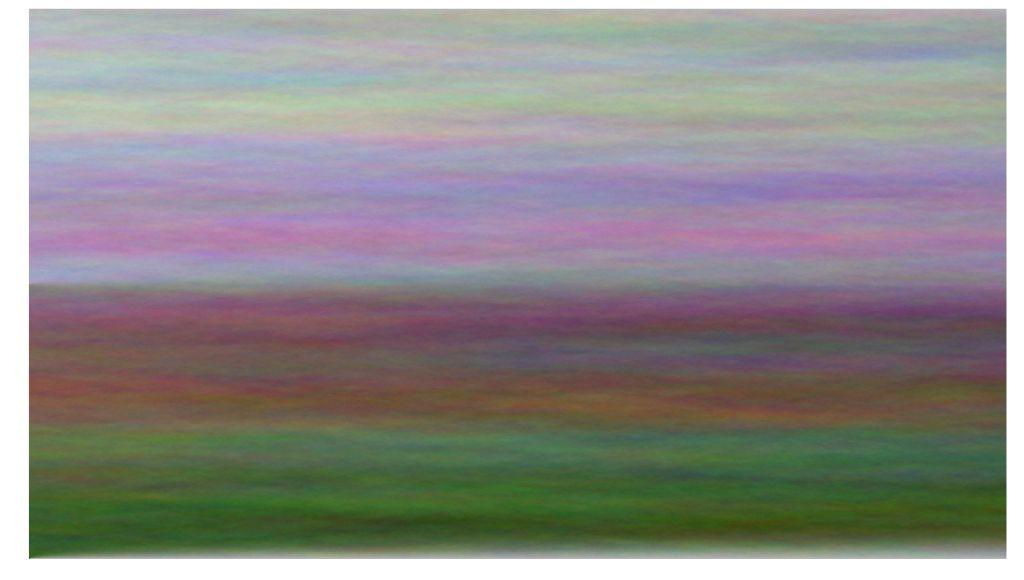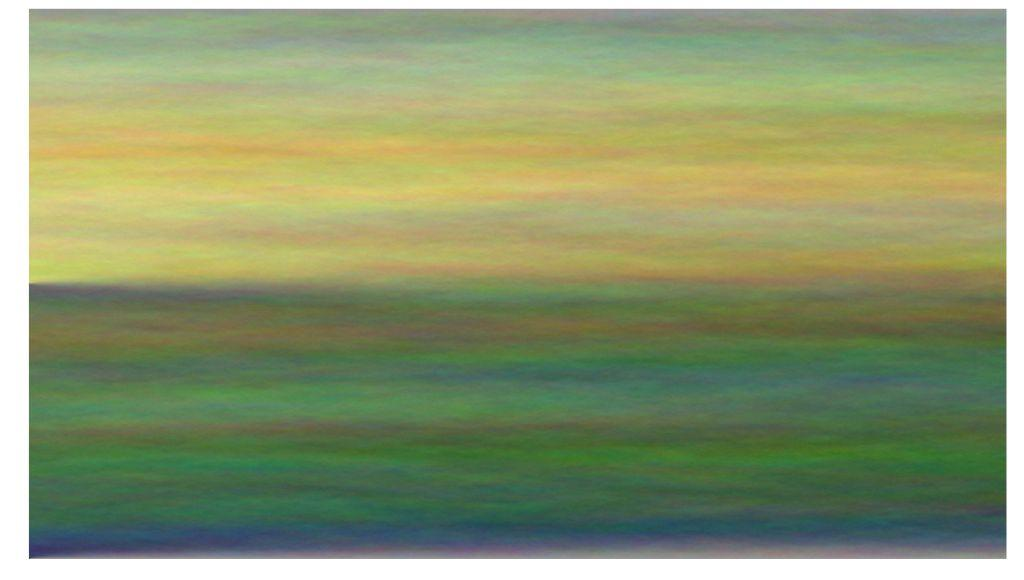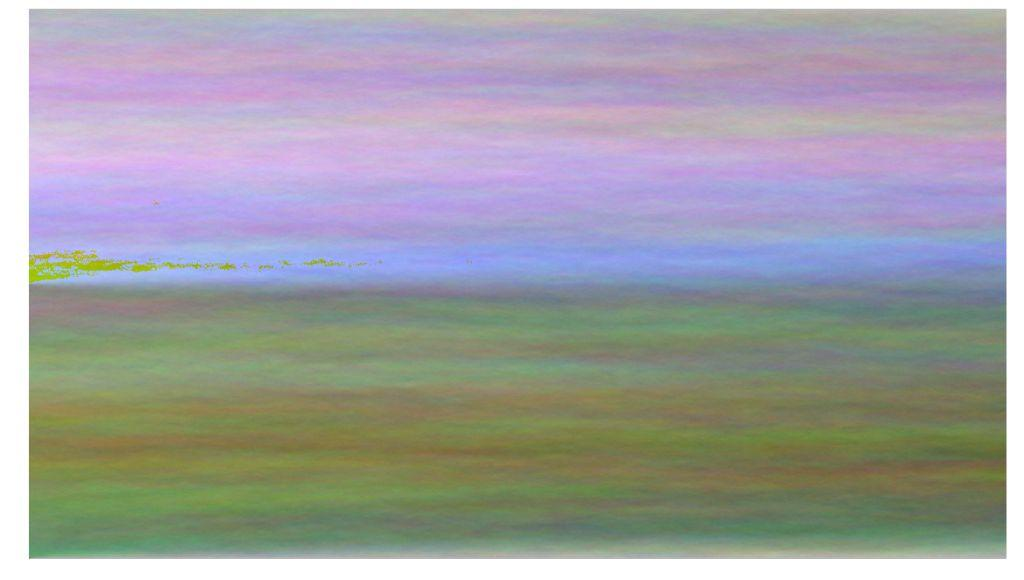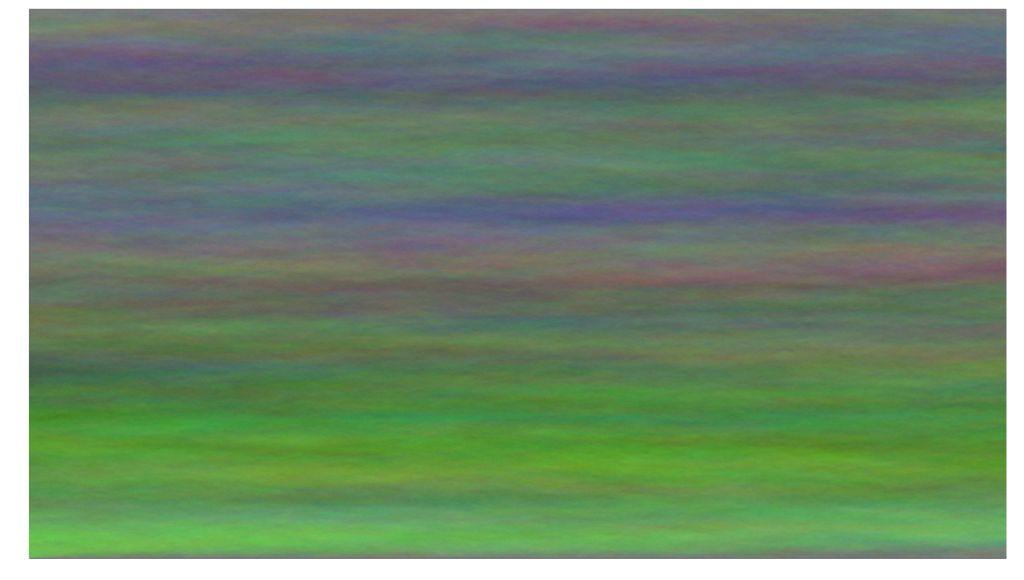.

.

.

.

.

# HealthyNumerics

HealthPoliticsEconomics | Quant Analytics | Numerics

# An accelerated generator of pastel canvas

We have found in this inspiring blog post a lean engine that generates smart pastel canvas.

The algorithm starts at the left boundary where the first column is initiated with a color that oscillates randomly around a certain value. The algorithm marches now through the canvas to the right. Each pixel within a new column is computed as the mean value of the three nearest neighbouring pixels of the left column - and a certain amount of random noise is added at this step.

The results are images with a lot of atmospheric flair.

# Vectorized code

We have modified the original code and replaced the two outer for-loops by vectorized forms. We use Python's slice-forms which allow for a lean writing of the array's indices and still give a fast access to the elements of the array. We have tested that earlier here.

Further we have split the initialisation into a lower and an upper part. So we get a landscape/atmosphere impression.

```import sys
import numpy as np
from PIL import Image
import matplotlib.pylab as plt
import matplotlib.colors as mclr
np.set_printoptions(linewidth=200, precision=3)

def draw_pastell(nx=900, ny=1600, CL=180, rshift=3):
nz=3
mid = nx//2
dCL = 50
#---- start the coloring ----------
A = np.ones((nx,ny,nz)) *CL           # initialize the image matrix
#np.random.seed(1234)                  # initialize RNG

#---- initialize the lower part ----
ix = slice(0,mid-1);   iz = slice(0,nz)  # color the left boundary
A[ix,0,iz] =  CL + np.cumsum(np.random.randint(-rshift, rshift+1, size=(mid-1,nz)),axis=0 )

#---- initialize the upper part ----
ix = slice(mid,nx);   iz = slice(0,nz)  # color the left boundary
A[ix,0,iz] =  CL-dCL + np.cumsum(np.random.randint(-rshift, rshift+1, size=(nx-mid,nz)),axis=0 )

#---- march to the right boundary -------------
ix = slice(1,nx-1); ixm = slice(0,nx-2); ixp = slice(2,nx)
for jy in range(1,ny):                # smear the color to the right boundary
A[ix,jy,iz] = 0.3333*(A[ixm,jy-1,iz] + A[ix,jy-1,iz] + A[ixp,jy-1,iz]) + np.random.randint(-rshift, rshift+1, size=(nx-2,nz))

#---- show&save grafics ---------
im = Image.fromarray(A.astype(np.uint8)).convert('RGBA')
fig, ax = plt.subplots(figsize=(20,14));
fileName = 'pic_pastell_B_{}_{}.png'.format(CL,rshift)
im.save(fileName)
plt.axis('off'); plt.imshow(im)

# nx, ny :   size of image (x,y)
# CL     :   color level
# rshift :   spread of random numbers
draw_pastell(nx=900, ny=1600, CL=181, rshift=3)
``````draw_pastell(nx=900, ny=1600, CL=151, rshift=3)
``````draw_pastell(nx=900, ny=1600, CL=183, rshift=3)
```# Code with for-loops

```def draw_pastell_FOR(width=1600, height=900, CL=180, rshift=3):
arr = np.ones((height, width,3)) * CL
np.random.seed(1234)

for y in range(1,height):
arr[y,0] = arr[y-1,0] + np.random.randint(-rshift, rshift+1, size=3)

for x in range(1, width):
for y in range(1,height-1):
arr[y,x] = ((arr[y-1, x-1] + arr[y,x-1] + arr[y+1,x-1])/3 ) + np.random.randint(-rshift, rshift+1, size=3)

im = Image.fromarray(arr.astype(np.uint8)).convert('RGBA')
filename = 'pic_pastell_FOR.png'
im.save(filename)
fig, ax = plt.subplots(figsize=(20,20));
plt.axis('off'); plt.imshow(im)

draw_pastell_FOR(width=1600, height=900, CL=120, rshift=3)
```# Comparing the speed

```%timeit draw_pastell(width=1600, hight=900, CL=180, rshift=3)
Result:
1.8 s ± 23.5 ms per loop (mean ± std. dev. of 7 runs, 1 loop each)

%timeit draw_pastell_FOR(width=1600, hight=900, CL=180, rshift=3)
Result:
22.6 s ± 202 ms per loop (mean ± std. dev. of 7 runs, 1 loop each)
```

The difference of the runtime is about a factor 12.